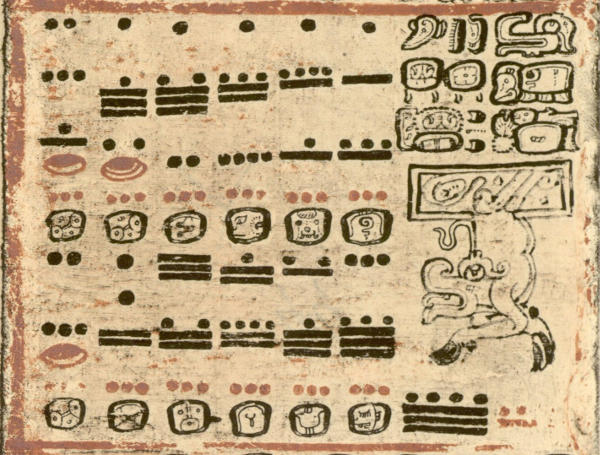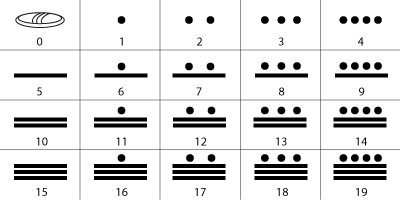# Maya Mathematics

The ancient Maya arithmetic and numeration system, with its dot-and-bar notation and concept of zero, is a fascinating topic. In this article I will explain how to read the Maya numerals and how to do mathematical operations in the Maya vigesimal system.Maya Numerals in the Dresden Codex

NB: specialists of the Maya civilisation say “Maya numerals”, “Maya number system”, “Maya mathematics” and not “Mayan numerals” etc. The adjective “Mayan” is used only in reference to languages.

Page content:

## Maya Mathematics: Introduction

The Maya were advanced mathematicians. Their numerical system, possibly one of the world’s most advanced at the time, allowed the Maya to do the elaborate calculations needed to make precise astronomical predictions. The precision of their observations along with their astronomical and calendrical recordkeeping were astonishingly accurate.

It now appears that the Maya had begun to use a system of numeration by position as early as the Late Preclassic (ca 400 BC-150 AD). Also called place-value notation, a positional notation is a system for representation of numbers -like our own- in which the value of a numeral symbol depends on its position (e.g. the “ones place”, “tens place”, “hundreds place”).

One of the great intellectual accomplishments of the Maya was the use of the mathematical concept of zero. They were only one of two ancient civilisations to use it (the great Egyptians never used the concept of zero), and the appearance of the zero in Maya inscriptions is one of the earliest known instances of this concept in the world.

Contrary to popular belief, due to geographical disconnect before the Conquest, Maya and Central America (Mesoamerica) never had any influence on -and reciprocally, had not been influenced by- Old World mathematics.

## How to Read Maya Numerals

In their numeral system, the ancient Maya only used three symbols to represent all numbers. A dot has a numerical value of 1, a line (or bar) a numerical value of 5 and a shell has the value of “completion” or 0. That’s the dot-and-bar notation used all over Mesoamerica.

These symbols (dot, bar and shell) are thought to represent items that the Maya people might have first used to count with, such as pebbles, sticks and shells.

In other words, zero is represented by a shell; 1 to 4 are represented by dots. The Maya wrote their numbers from top to bottom rather than from left to right. Multiples of five are represented by lines, with extra dots being added to complete the numbers as shown below:Maya Numerals

The numbers above nineteen are indicated on the basis of their vertical position. The Maya used a vigesimal (Base-20) system, so each position is a power of twenty.

## A Vigesimal System

Our own Hindu-Arabic numeral system uses powers of tens (“ones place”, “tens place”, “hundreds place”), it is a decimal or Base-10 system. The ancient Maya used a vigesimal (Base-20) notation in which each position is a power of twenty.

In a Base-10 system, there are 9 digits (1, 2, 3, 4, 5, 6, 7, 8, 9) plus a zero. When writing numbers, once we get to ‘9’ we then have to move across to the next column. We write a ‘one’ followed by a ‘zero’ to show that we have moved across. Zero is a ‘place-holder’. From there, we will use the 9 numerals to represent the number we want up to 99. Then, when we go beyond ’99’, we move across to the next column and write ‘100’.

So, for example, in our decimal system, 39 is ‘3 x 10’ and ‘9 x 1’

The Maya used a similar system using their 19 numerals and then moving to the next section and putting a zero (represented by the shell) as a placeholder. Another difference is that the Maya used rows instead of columns, starting from the bottom and working upwards. So the place values were multiples of 20s: 1s, 20s (20 x 1), 400s, (20 x 20), 8,000s (20 x 400) and so on.

Using the previous example, ’39’ would be written as follows in the Maya system:

## How to Convert Maya Numbers to Base-10 Numbers

In the decimal system ‘815’ is:

And now in the Maya vigesimal system:

And now, let us try a bigger number:

The Maya vigesimal system is every bit as useful and efficient as our own decimal-place system. Besides being base 20 instead of base 10, it differs from ours in using combinations of just three symbols (shell, bar, dot), whereas we have to use 10 different symbols (0, 1, 2, 3, 4, 5, 6, 7, 8, 9).

## How to Convert Base-10 Numbers to Maya Numbers

To figure out the Maya equivalent of a number from our decimal system, you need to divide the number into powers of twenty (8000, 400, 20). Let’s take 4285, for example:  4285 = (10×20²) + (14×20¹) + 5

How did we get there? We know that 4285 is smaller than 8000 (20³) so we’re going to divide it by 400 (20²) which gives us ’10’. The remainder is 285 which we’re going to divide by 20 (20¹). That gives us ’14’ plus ‘5’ units left over.

Here is another way to calculate a Maya number:

## How to Calculate with Maya Numbers: Adding and Subtracting

Adding and subtracting numbers using the Maya numeral system is quite simple.

### How to add Maya numbers

Addition is performed by combining the numeric symbols at each level.

### How to subtract Maya numbers

Similarly with subtraction, simply remove the numeric symbols.

## Try Yourself

What number is being shown in each of the images? (You will find the answers at the bottom of the page).

## Further Resources on Maya Arithmetic

• Maya Codices – by Gabrielle Vail and Christine Hernández. This site features a searchable translation and analysis of the four remaining Maya codices, including the Dresden Codex (screenfold books), painted by the Maya scribes before the Spanish conquest in the early 16th century. The codices contain information about Maya beliefs and rituals, as well as everyday activities, all framed within an astronomical and calendrical context.

Answers to test above – a: 806, b: 2005

© 2020 Maya Achaeologist | All Rights Reserved | Manage consent